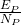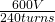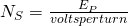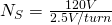Main Body

# Operation (How the heck do they work?)

## It’s Time for Some Truth

• The input side is always the primary winding. This is the side that is always hooked up to the source of voltage.

• The output side is always the secondary winding. This is the side that is always hooked up to the load.

• H leads are high-voltage leads. They have a lower gauge wire with larger insulation.

• X leads are low-voltage leads. They have a thicker gauge wire with smaller insulation.

## Key Takeaways

A lot of examples are step-down transformers, but this is not always the case. Keep this in mind. Your H leads are not always your primary!

X1 and H1 will always have the same polarity.

## Voltage

When the primary is energized from an AC supply with no load on the secondary, it acts as an inductor.

Self-inductance creates a CEMF to limit the current to 2%–5% of the primary full-load current. This small amount of current is called exciting current (also known as magnetizing current).

The secondary voltage depends on primary voltage and turns, and the secondary turns. The ratio between primary and secondary voltage is the same as the ratio between primary and secondary turns.

Which means:

Primary volts per turn = secondary volts per turn

Example #1

What are the volts per turn of a transformer with a voltage rating of
600V–20V if the high-voltage winding contains 240 turns?== 2.5 volts per turn

Example #2

How many turns would there be in the low-voltage winding of the transformer in question 1?N= 48 turns

When it comes to using volts/turn, it’s good to remember that volts/turn on the primary is equal to the volts/turn on the secondary but it can sometimes be confusing to use this in calculations. An easier method is to use the turns ratio method.

If you take the higher number of turns and divide it by the lower number of turns, you get a ratio.  For example:

A transformer with 100 turns on the primary and 50 turns on the secondary will have a turns ratio of 2:1. Therefore if 120 volts is on the primary, then 60 volts will be impressed on the secondary.

This video walks through and explains how to use the turns ratio to calculate the voltage on the primary or the secondary.

## Current

The easiest way to calculate current is to use the turns ratio as well.

The only difference is that higher turns means lower current, so you will be using the turns ratio backwards.

Example #3

A transformer has 600 turns on the primary and 120 turns on the secondary. 300 volts is impressed on the primary and a current of 40 amps circulates on the secondary. Calculate:

1. Turns ratio
2. Secondary voltage
3. Primary current

600/120 = 5:1 turns ratio

300 V/5 (Ratio) = 60 volts on the secondary

4 amps/5(Ratio) = 8 amps on the primary

This video walks through how to use the turns ratio to calculate current. It also shows another method that many find easier. In a transformer, the power input is always equal to the power output.

## Rated vs. Actual

Transformer windings are designed to be able to handle a certain amount of voltage and a certain amount of current.

This is where its VA rating comes from.

A transformer is rated 1000 VA, 100V/10V, and is connected for step-down operation. Therefore:

Rated Ip= 10 A

Rated Is= 100 A

Rated VP= 100 V

Rated VS= 10 V

This is what the transformer is designed to handle—this is its maximum. Anything over this will burn out the windings.

If we add a 5 Ω resistor load to the secondary, we can calculate Ip and Is.

Actual Ip= 0.2 A

Actual Is= 2 A

This transformer is delivering 20 VA, not 1000 VA.

The same holds true for the voltage.

If we put 50 V on the primary, we would get a secondary voltage of 5 V.

Remember that the rated values are the maximum values that the windings can see. We are given the VA and the V, and we use these values to determine the maximum current.

As long as those values are not exceeded, we can use the transformer for our purposes.